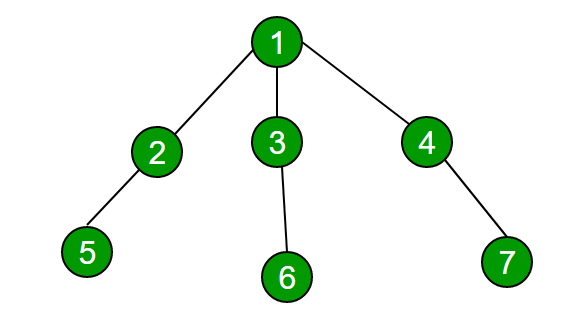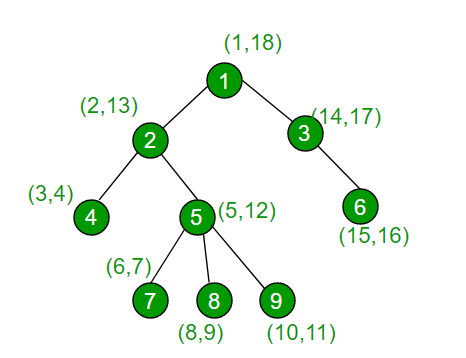GeeksforGeeks App
Open AppBrowser
Continue

# Check if two nodes are on same path in a tree

Given a tree (not necessarily a binary tree) and a number of queries such that every query takes two nodes of the tree as parameters. For every query pair, find if two nodes are on the same path from the root to the bottom.

For example, consider the below tree, if given queries are (1, 5), (1, 6), and (2, 6), then answers should be true, true, and false respectively.Note that 1 and 5 lie on the same root to leaf path, so do 1 and 6, but 2 and 6 are not on the same root to leaf path.

It is obvious that the Depth First Search technique is to be used to solve the above problem, the main problem is how to respond to multiple queries fast. Here our graph is a tree which may have any number of children. Now DFS in a tree, if started from root node, proceeds in a depth search manner i.e. Suppose root has three children and those children have only one child with them so if DFS is started then it first visits the first child of root node then will go deep to the child of that node. The situation with a small tree can be shown as follows:

The order of visiting the nodes will be – 1 2 5 3 6 4 7 .
Thus, other children nodes are visited later until completely one child is successfully visited till depth. To simplify this if we assume that we have a watch in our hand, and we start walking from the root in DFS manner.

Intime – When we visit the node for the first time
Outtime- If we again visit the node later but there are no children unvisited we call it outtime,

Note: Any node in its sub-tree will always have intime < its children (or children of children) because it is always visited first before children (due to DFS) and will have outtime > all nodes in its sub-tree because before noting the outtime it waits for all of its children to be marked visited.

For any two nodes u, v if they are in the same path then,

```Intime[v] < Intime[u] and Outtime[v] > Outtime[u]
OR
Intime[u] < Intime[v] and Outtime[u ]> Outtime[v]```
• If a given pair of nodes follows any of the two conditions, then they are on the same root to the leaf path.
• Else not on the same path (If two nodes are on different paths it means that no one is in the subtree of each other).

Pseudo Code

We use a global variable time which will be incremented as dfs for a node begins and will also be incremented after

```DFS(v)
increment timer
Intime[v] = timer
mark v as visited
for all u that are children of v
DFS(u)
increment timer
Outtime[v] = timer
end```

Time Complexity – O(n) for preprocessing and O(1) per query.

Implementation:
Below is the implementation of the above pseudo-code.

## C++

 `// C++ program to check if given pairs lie on same``// path or not.``#include``using` `namespace` `std;``const` `int` `MAX = 100001;` `// To keep track of visited vertices in DFS``bool` `visit[MAX] = {0};` `// To store start and end time of all vertices``// during DFS.``int` `intime[MAX];``int` `outtime[MAX];` `// initially timer is zero``int` `timer = 0;` `// Does DFS of given graph and fills arrays``// intime[] and outtime[]. These arrays are used``// to answer given queries.``void` `dfs(vector<``int``> graph[], ``int` `v)``{``    ``visit[v] = ``true``;` `    ``// Increment the timer as you enter``    ``// the recursion for v``    ``++timer;` `    ``// Upgrade the in time for the vertex``    ``intime[v] = timer;` `    ``vector<``int``>::iterator it = graph[v].begin();``    ``while` `(it != graph[v].end())``    ``{``        ``if` `(visit[*it]==``false``)``            ``dfs(graph, *it);``        ``it++;``    ``}` `    ``// increment the timer as you exit the``    ``// recursion for v``    ``++timer;` `    ``// upgrade the outtime for that node``    ``outtime[v] = timer;``}` `// Returns true if 'u' and 'v' lie on same root to leaf path``// else false.``bool` `query(``int` `u, ``int` `v)``{``    ``return` `( (intime[u]outtime[v]) ||``             ``(intime[v]outtime[u]) );``}` `// Driver code``int` `main()``{``    ``// Let us create above shown tree``    ``int` `n = 9; ``// total number of nodes``    ``vector<``int``> graph[n+1];``    ``graph.push_back(2);``    ``graph.push_back(3);``    ``graph.push_back(6);``    ``graph.push_back(4);``    ``graph.push_back(5);``    ``graph.push_back(7);``    ``graph.push_back(8);``    ``graph.push_back(9);` `    ``// Start dfs (here root node is 1)``    ``dfs(graph, 1);` `    ``// below are calls for few pairs of nodes``    ``query(1, 5)? cout << ``"Yes\n"` `: cout << ``"No\n"``;``    ``query(2, 9)? cout << ``"Yes\n"` `: cout << ``"No\n"``;``    ``query(2, 6)? cout << ``"Yes\n"` `: cout << ``"No\n"``;` `    ``return` `0;``}`

## Java

 `// Java program to check if given``// pairs lie on same path or not.``import` `java.util.*;` `class` `GFG{``    ` `static` `int` `MAX = ``100001``;` `// To keep track of visited vertices in DFS``static` `boolean` `[]visit = ``new` `boolean``[MAX];` `// To store start and end time of all vertices``// during DFS.``static` `int` `[]intime = ``new` `int``[MAX];``static` `int` `[]outtime = ``new` `int``[MAX];` `// Initially timer is zero``static` `int` `timer = ``0``;` `// Does DFS of given graph and fills arrays``// intime[] and outtime[]. These arrays are used``// to answer given queries.``static` `void` `dfs(Vector graph[], ``int` `v)``{``    ``visit[v] = ``true``;` `    ``// Increment the timer as you enter``    ``// the recursion for v``    ``++timer;` `    ``// Upgrade the in time for the vertex``    ``intime[v] = timer;` `    ``for``(``int` `it : graph[v])``    ``{``        ``if` `(visit[it] == ``false``)``            ``dfs(graph, it);``            ` `        ``it++;``    ``}` `    ``// Increment the timer as you exit the``    ``// recursion for v``    ``++timer;` `    ``// Upgrade the outtime for that node``    ``outtime[v] = timer;``}` `// Returns true if 'u' and 'v' lie on``// same root to leaf path else false.``static` `boolean` `query(``int` `u, ``int` `v)``{``    ``return` `((intime[u] < intime[v] &&``            ``outtime[u] > outtime[v]) ||``            ``(intime[v] < intime[u] &&``            ``outtime[v] > outtime[u]));``}` `// Driver code``public` `static` `void` `main(String[] args)``{``    ` `    ``// Let us create above shown tree``    ``int` `n = ``9``; ``// total number of nodes``    ` `    ``@SuppressWarnings``(``"unchecked"``)``    ``Vector []graph = ``new` `Vector[n + ``1``];``    ``for``(``int` `i = ``0``; i < graph.length; i++)``        ``graph[i] = ``new` `Vector();``        ` `    ``graph[``1``].add(``2``);``    ``graph[``1``].add(``3``);``    ``graph[``3``].add(``6``);``    ``graph[``2``].add(``4``);``    ``graph[``2``].add(``5``);``    ``graph[``5``].add(``7``);``    ``graph[``5``].add(``8``);``    ``graph[``5``].add(``9``);` `    ``// Start dfs (here root node is 1)``    ``dfs(graph, ``1``);` `    ``// Below are calls for few pairs of nodes``    ``if` `(query(``1``, ``5``))``        ``System.out.print(``"Yes\n"` `);``    ``else``        ``System.out.print(``"No\n"``);``        ` `    ``if` `(query(``2``, ``9``))``        ``System.out.print(``"Yes\n"``);``    ``else``        ``System.out.print(``"No\n"``);``    ` `    ``if` `(query(``2``, ``6``))``        ``System.out.print(``"Yes\n"` `);``    ``else``        ``System.out.print(``"No\n"``);``}``}` `// This code is contributed by Princi Singh`

## Python3

 `# contributed by saurabh_jain861``# Python3 program to check if given``# pairs lie on same path or not.` `# Does DFS of given graph and fills``# arrays intime[] and outtime[].``# These arrays are used to answer``# given queries.``def` `dfs(graph, v):``    ``global` `intime, outtime, visit, ``MAX``, timer``    ``visit.add(v)` `    ``# Increment the timer as you enter``    ``# the recursion for v``    ``timer ``+``=` `1` `    ``# Upgrade the in time for the vertex``    ``intime[v] ``=` `timer``    ``it ``=` `0``    ``while` `it < ``len``(graph[v]):``        ``if` `(graph[v][it] ``not` `in` `visit):``            ``dfs(graph, graph[v][it])``        ``it ``+``=` `1` `    ``# increment the timer as you``    ``# exit the recursion for v``    ``timer ``+``=` `1` `    ``# upgrade the outtime for that node``    ``outtime[v] ``=` `timer` `# Returns true if 'u' and 'v' lie on``# same root to leaf path else false.``def` `query(u, v):``    ``global` `intime, outtime, visit, ``MAX``, timer``    ``return` `((intime[u] < intime[v] ``and``             ``outtime[u] > outtime[v]) ``or``            ``(intime[v] < intime[u] ``and``             ``outtime[v] > outtime[u]) )` `# Driver code``MAX` `=` `100001` `# To keep track of visited vertices in DFS``visit ``=``set``()` `# To store start and end time of``# all vertices during DFS.``intime ``=` `[``0``] ``*` `MAX``outtime ``=` `[``0``] ``*` `MAX` `# initially timer is zero``timer ``=` `0` `# Let us create above shown tree``n ``=` `9` `# total number of nodes``graph ``=` `[[] ``for` `i ``in` `range``(n``+``1``)]``graph[``1``].append(``2``)``graph[``1``].append(``3``)``graph[``3``].append(``6``)``graph[``2``].append(``4``)``graph[``2``].append(``5``)``graph[``5``].append(``7``)``graph[``5``].append(``8``)``graph[``5``].append(``9``)` `# Start dfs (here root node is 1)``dfs(graph, ``1``)` `# below are calls for few pairs of nodes``print``(``"Yes"``) ``if` `query(``1``, ``5``) ``else` `print``(``"No"``)``print``(``"Yes"``) ``if` `query(``2``, ``9``) ``else` `print``(``"No"``)``print``(``"Yes"``) ``if` `query(``2``, ``6``) ``else` `print``(``"No"``)` `# This code is contributed by PranchalK`

## C#

 `// C# program to check if given``// pairs lie on same path or not.``using` `System;``using` `System.Collections.Generic;``class` `GFG{``    ` `static` `int` `MAX = 100001;` `// To keep track of visited``// vertices in DFS``static` `bool` `[]visit =``       ``new` `bool``[MAX];` `// To store start and end``// time of all vertices``// during DFS.``static` `int` `[]intime =``       ``new` `int``[MAX];``static` `int` `[]outtime =``       ``new` `int``[MAX];` `// Initially timer is zero``static` `int` `timer = 0;` `// Does DFS of given graph``// and fills arrays intime[]``// and outtime[]. These arrays``// are used to answer given queries.``static` `void` `dfs(List<``int``> []graph,``                ``int` `v)``{``  ``visit[v] = ``true``;` `  ``// Increment the timer as``  ``// you enter the recursion``  ``// for v``  ``++timer;` `  ``// Upgrade the in time``  ``// for the vertex``  ``intime[v] = timer;` `  ``foreach``(``int` `it ``in` `graph[v])``  ``{``    ``if` `(visit[it] == ``false``)``      ``dfs(graph, it);``  ``}` `  ``// Increment the timer as``  ``// you exit the recursion for v``  ``++timer;` `  ``// Upgrade the outtime for``  ``// that node``  ``outtime[v] = timer;``}` `// Returns true if 'u' and``// 'v' lie on same root to``// leaf path else false.``static` `bool` `query(``int` `u,``                  ``int` `v)``{``  ``return` `((intime[u] < intime[v] &&``           ``outtime[u] > outtime[v]) ||``          ``(intime[v] < intime[u] &&``           ``outtime[v] > outtime[u]));``}` `// Driver code``public` `static` `void` `Main(String[] args)``{   ``  ``// Let us create above shown tree``  ``// total number of nodes``  ``int` `n = 9;` `  ``List<``int``> []graph =``       ``new` `List<``int``>[n + 1];``  ` `  ``for``(``int` `i = 0;``          ``i < graph.Length; i++)``    ``graph[i] = ``new` `List<``int``>();` `  ``graph.Add(2);``  ``graph.Add(3);``  ``graph.Add(6);``  ``graph.Add(4);``  ``graph.Add(5);``  ``graph.Add(7);``  ``graph.Add(8);``  ``graph.Add(9);` `  ``// Start dfs (here root``  ``// node is 1)``  ``dfs(graph, 1);` `  ``// Below are calls for few``  ``// pairs of nodes``  ``if` `(query(1, 5))``    ``Console.Write(``"Yes\n"` `);``  ``else``    ``Console.Write(``"No\n"``);` `  ``if` `(query(2, 9))``    ``Console.Write(``"Yes\n"``);``  ``else``    ``Console.Write(``"No\n"``);` `  ``if` `(query(2, 6))``    ``Console.Write(``"Yes\n"` `);``  ``else``    ``Console.Write(``"No\n"``);``}``}` `// This code is contributed by Amit Katiyar`

Output

```Yes
Yes
No```

Illustration:
From the diagram below to understand more, we can have some examples. DFS algorithm as modified above will result in the following intime and outtime for the vertex of the tree as labeled there. Now we will consider all the cases.Case 1: Nodes 2 and 4: Node 2 has intime less than node 4 but since 4 is in its sub tree so it will have a greater exit time than 4 . Thus, condition is valid and both are on the same path.
Case 2: Nodes 7 and 6: Node 7 has intime less than node 6 but since both nodes are not in each other’s sub tree so their exit time does not follow the required condition.

This article is contributed by Saurabh Joshi. If you like GeeksforGeeks and would like to contribute, you can also write an article and mail your article to contribute@geeksforgeeks.org. See your article appearing on the GeeksforGeeks main page and help other Geeks.